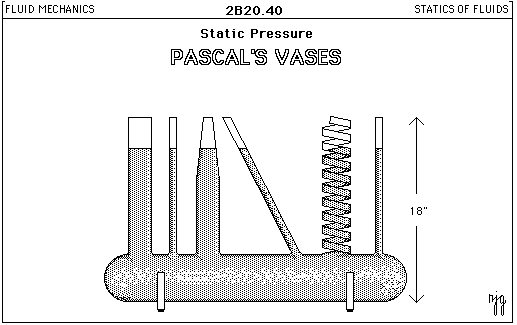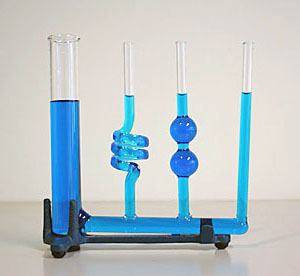# Pressure liquid

what would the pressure of liquid at a depth be in a container which is slanted?

i suspect $$h.d.g.sinAngle$$. correct me if i am wrong

in the derivation of the pressure of liquid, the weight is assumed to act perpendicular to the column of liquid. Plz comment

russ_watters
Mentor
Gravity pulls straight down, so...

of course but i find similar case to the inclined plane. can somebody give a reasoning?

russ_watters
Mentor
What do you find similar about an inclined plane? What is YOUR reasoning?

Doc Al
Mentor
The pressure depends on the depth beneath the surface, which is not slanted. As already stated, the shape of the container--whether slanted or vertical--is irrelevant.

If you want more, give a specific example of what you have in mind with a diagram.

russ_watters
Mentor
Sorry, we don't spoonfeed here. If you want to learn/want help, you need to show some effort at trying to figure it out for yourself. Then when you make a wrong turn, we'll nudge you back in the right direction.

wouldnt this imply that the liquid would accelerate at g in slanted tubes?

Doc Al
Mentor
wouldnt this imply that the liquid would accelerate at g in slanted tubes?
No. Please describe exactly what you have in mind. Are you talking about hydrostatic pressure? (Which is what I assumed.) Or fluid dynamics?

russ_watters
Mentor
g is acceleration due to gravity. If you block that acceleration, the required force is f=mg. Aka, weight.

DaveC426913
Gold Member
wouldnt this imply that the liquid would accelerate at g in slanted tubes?
Since you're talking about pressure-at-depth then you're talking about a container full of fluid (as opposed to, say, an air-filled container with an amount of water placed in it).

Water suspended in water is neutrally buoyant, so its not like some arbitrary mass of water is going to start sliding to the bottom, accelerating under gravity.

No. Please describe exactly what you have in mind. Are you talking about hydrostatic pressure? (Which is what I assumed.) Or fluid dynamics?

I am not familiar with the terms but i guess you are asking whether i am talking about stationary fluids or flowing ones. Eg. in an inclined plane, there is a mass a top, even though its weight acts exactly downward, it would rather move along the plane. And the force with with it moves along the plane is lesser according to its slope. Same for liquids. But as dave said while the liquid is continuous and stationary, the force with with a finite upper part of liquid exerts on the lower part will be the same as for the case of liquids in vertical column. This is not clear to me.

Eg. lets take a column of liquid standing upright and pour some water into it. And then slant it a bit. Then the depth of the liquid increases even though it is not continuous on the upper part (i hope this is understood). So as the depth increases although not uniformly, the pressure in one side must increase. Help me out with this.

D H
Staff Emeritus
Pascal's vases:Another set:Notice that no matter how weird the shape, the tops of the liquid surfaces in the different containers are at the same level. The pressure difference in some container from bottom to top does not depend on shape. It depends only the height of the liquid.

i havent learned any of hydrodynamics or hydrostatics. So i think this phenomenon is taken as as true. Is there a proof for it?

D H
Staff Emeritus
The diagram and image (real) I posted in [post=3643843]post #16[/post] are pretty solid evidence. Hydraulic pumps rely on this principle.

russ_watters
Mentor
i havent learned any of hydrodynamics or hydrostatics.
This is getting very frustrating for me because a bunch of people are putting a bunch of effort into helping you learn, but it doesn't seem like you are trying at all. For example, you said you don't know terms like "hydrostatic", but that term is defined in the very first sentence of the link i gave you in the first reply!

"Learning" is not something we can give you: we can tell you where to find the knowledge, but you have to get it into your head.

i suspect $$h.d.g.sinAngle$$. correct me if i am wrong

OP , when I was a kid (well now also I am a kid in class 10th !) I also used to think the same way as you are currently.

I used to say :
Pressure = (Force x sin θ)/Area

Now I know that I was wrong because - Pressure is "thrust upon area." It is defined this way. Thrust is defined as force acting perpendicularly on a body.

Hence always ,

P=hρg x sin 90o or P = hdg x cos 0o

As sin 90o = cos 0o = 1

So P=hρg

Do you get it now ?

the diagram is clear, and i asked the question, and i had seen before, so i asked the question. to, sankalpmittal
take a glass of water, then slant it slightly so as not to drop the water, As the depth increases on one side, doesent the pressure increase?

so the pressure depends on the vertical distance of he column irrespective of the shape. Am i correct?

Doc Al
Mentor
so the pressure depends on the vertical distance of he column irrespective of the shape. Am i correct?
Yes. Pressure depends on the vertical distance below the fluid surface. Shape of the container has nothing to do with it.

Whether the pressure increases or not depends on the shape of the object and where you measure the pressure. When you have a rectangular container with 1x1x1 m dimensions and you have 1 cm of water on the bottom, and when you turn the container at a 45 degree angle, the water will accumulate in one corner and the height from the bottom of the container to the water level will be higher than before, therefore also the pressure on the bottom of the container. However, if you have a narrow tube and a lot of water in it, 10 m tall and 1 cm wide, with 2 m of water in it, if you then turn it at an angle the water level from the bottom of the container will be less, meaning the pressure on the bottom would also be less.

So to find out whether or not the pressure will increase, you will have to calculate the water level when straight and the water level when slanted. Then you can compare these to find the pressure difference.

thank u chingel, thats what i wanted.